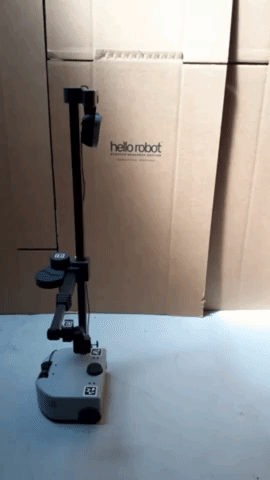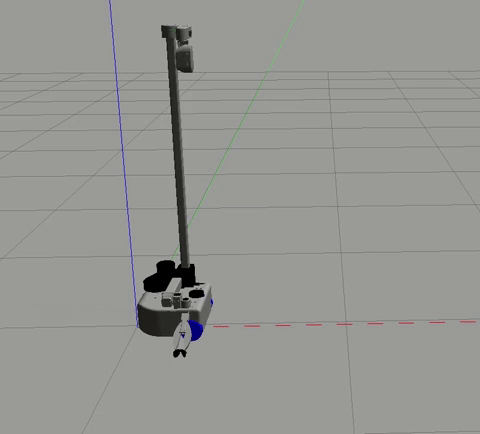# Example 1

## Example 1The goal of this example is to give you an enhanced understanding of how to control the mobile base by sending `Twist` messages to a Stretch robot.

Begin by running the following command in a new terminal.

``````# Terminal 1
roslaunch stretch_core stretch_driver.launch
``````

Switch the mode to navigation mode using a rosservice call. Then drive the robot forward with the move.py node.

``````# Terminal 2
cd catkin_ws/src/stretch_tutorials/src/
python move.py
``````
To stop the node from sending twist messages, type Ctrl + c.

### The Code

Below is the code which will send Twist messages to drive the robot forward.

``````#!/usr/bin/env python

import rospy
from geometry_msgs.msg import Twist

class Move:
"""
A class that sends Twist messages to move the Stretch robot forward.
"""
def __init__(self):
"""
Function that initializes the subscriber.
:param self: The self reference.
"""
self.pub = rospy.Publisher('/stretch/cmd_vel', Twist, queue_size=1) #/stretch_diff_drive_controller/cmd_vel for gazebo

def move_forward(self):
"""
Function that publishes Twist messages
:param self: The self reference.

:publishes command: Twist message.
"""
command = Twist()
command.linear.x = 0.1
command.linear.y = 0.0
command.linear.z = 0.0
command.angular.x = 0.0
command.angular.y = 0.0
command.angular.z = 0.0
self.pub.publish(command)

if __name__ == '__main__':
rospy.init_node('move')
base_motion = Move()
rate = rospy.Rate(10)
while not rospy.is_shutdown():
base_motion.move_forward()
rate.sleep()
``````

### The Code Explained

Now let's break the code down.

``````#!/usr/bin/env python
``````
Every Python ROS Node will have this declaration at the top. The first line makes sure your script is executed as a Python script.

``````import rospy
from geometry_msgs.msg import Twist
``````
You need to import `rospy` if you are writing a ROS Node. The `geometry_msgs.msg` import is so that we can send velocity commands to the robot.

``````class Move:
def __init__(self):
self.pub = rospy.Publisher('/stretch/cmd_vel', Twist, queue_size=1)#/stretch_diff_drive_controller/cmd_vel for gazebo
``````
This section of code defines the talker's interface to the rest of ROS. `pub = rospy.Publisher("/stretch/cmd_vel", Twist, queue_size=1)` declares that your node is publishing to the /stretch/cmd_vel topic using the message type `Twist`. The `queue_size` argument limits the amount of queued messages if any subscriber is not receiving them fast enough.

``````command = Twist()
``````
Make a `Twist` message. We're going to set all of the elements, since we can't depend on them defaulting to safe values.

``````command.linear.x = 0.1
command.linear.y = 0.0
command.linear.z = 0.0
``````
A `Twist` data structure has three linear velocities (in meters per second), along each of the axes. For Stretch, it will only pay attention to the x velocity, since it can't directly move in the y direction or the z direction.

``````command.angular.x = 0.0
command.angular.y = 0.0
command.angular.z = 0.0
``````
A `Twist` message also has three rotational velocities (in radians per second). The Stretch will only respond to rotations around the z (vertical) axis.

``````self.pub.publish(command)
``````
Publish the `Twist` commands in the previously defined topic name /stretch/cmd_vel.

``````rospy.init_node('move')
base_motion = Move()
rate = rospy.Rate(10)
``````
The next line, `rospy.init_node(NAME, ...)`, is very important as it tells rospy the name of your node -- until rospy has this information, it cannot start communicating with the ROS Master. NOTE: the name must be a base name, i.e. it cannot contain any slashes "/".

The `rospy.Rate()` function creates a Rate object. With the help of its method `sleep()`, it offers a convenient way for looping at the desired rate. With its argument of 10, we should expect to go through the loop 10 times per second (as long as our processing time does not exceed 1/10th of a second!)

``````while not rospy.is_shutdown():
base_motion.move_forward()
rate.sleep()
``````
This loop is a fairly standard rospy construct: checking the `rospy.is_shutdown()` flag and then doing work. You have to check `is_shutdown()` to check if your program should exit (e.g. if there is a Ctrl-C or otherwise). The loop calls `rate.sleep()`, which sleeps just long enough to maintain the desired rate through the loop.

## Move Stretch in SimulationUsing your preferred text editor, modify the topic name of the published `Twist` messages. Please review the edit in the move.py script below.

``````self.pub = rospy.Publisher('/stretch_diff_drive_controller/cmd_vel', Twist, queue_size=1)
``````

After saving the edited node, bringup Stretch in the empty world simulation. To drive the robot with the node, type the following in a new terminal

``````cd catkin_ws/src/stretch_tutorials/src/
python move.py
``````
To stop the node from sending twist messages, type Ctrl + c.